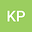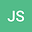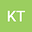Coefficient bounds in the class of functions associated with $q$-function theory
•••• Krzysztof Piejko,
• Janusz Sokół,
• Katarzyna Trabka-Wieclaw
Krzysztof Piejko
Rzeszow University of Technology
Author ProfileJanusz Sokół
University of Rzeszow
Author ProfileKatarzyna Trabka-Wieclaw
Lublin University of Technology
Author Profile## Abstract

In this paper, we use the concept of $q$-calculus in geometric function theory. For some $\alpha$, $\alpha\in [0,1)$, we consider normalized analytic functions $f$ such that $f’(z)/{\rm d}_qf(z)$ lies in half-plane $\{w:\mathfrak {Re}\ w>\alpha\}$ for all $z$, $|z|< 1$. Here ${\rm d}_q$ is the Jackson $q$-derivative operator well-known in the $q$-calculus theory. The paper is devoted to the coefficient problems of such functions for real and for complex numbers $q$. Coefficient bounds are of particular interest, because of them some geometrical properties of the function can be obtained.

#### Peer review status:UNDER REVIEW

28 Jun 2021Submitted to Mathematical Methods in the Applied Sciences
28 Jun 2021Assigned to Editor
28 Jun 2021Submission Checks Completed
15 Jul 2021Reviewer(s) Assigned